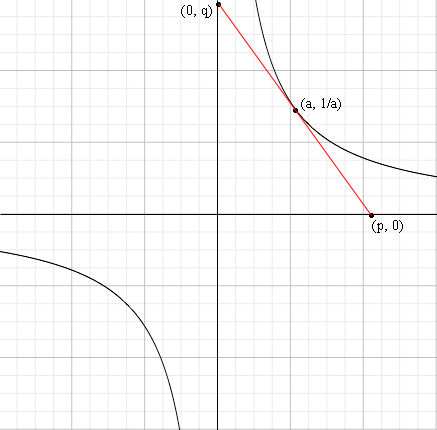SEARCH HOMEMath Central Quandaries & QueriesQuestion from Nirmala: Given that y=1/x, x is not equal to zero. Prove that the area of the triangle formed by the tangent and the coordinates axes is 2.Hi Nirmala,Use calculus to find the slope of the tangent to y = 1/x at the point (a, 1/a). Write the equation of the tangent line. Solve for p and q. Find the area of the triangle.

HarleyMath Central is supported by the University of Regina and The Pacific Institute for the Mathematical Sciences.